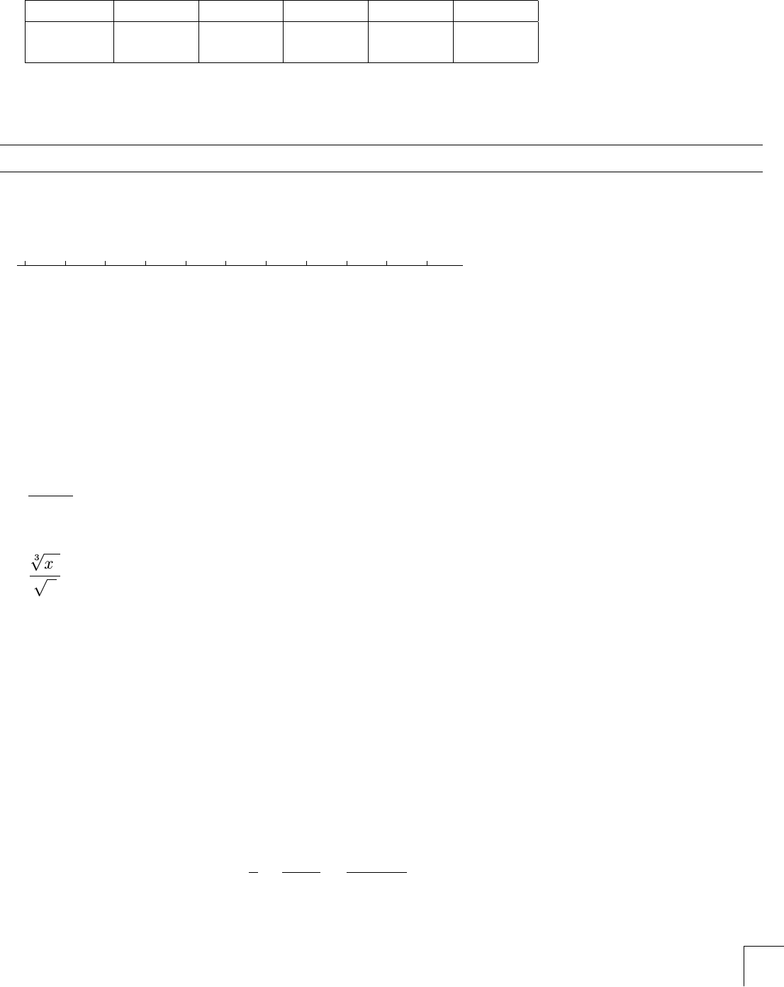# MATH 211 NIU 04Fall Solution

27 views5 pages
School
Department
Course
Professorpg score
Name:
Recitation Instructor: Recitation Day & Time:
Total/200 Page1/40 Page2/40 Page3/40 Page4/40 Page5/40
MATH 100 College Algebra
Final Exam
7:00–8:50pm, Wednesday May 14, 2003
Show All Your Work. Check that you have all five pages.
1. (10 points) Solve the inequality |2x1| ≥ 5.Graph your solution below.
(Remember to indicate clearly whether end-points are included or excluded).
x54321 0 1 2 3 4 5
2. (10 points) Simplify, assuming x, y positive. In the answer only positive rational exponents (no radicals)
should occur.
(a) (xy2)3
x2y3
(b)
3
x5
x
3. (10 points)
(a) Find the slope of the line through the points (2,3) and (0,3).
(b) Give the slope-intercept form of the equation of the straight line through the points in (a).
4. (10 points) Solve the equation 7
x3
x1=5
x(x1).
1
Unlock document

This preview shows pages 1-2 of the document.
Unlock all 5 pages and 3 million more documents.pg score
5. (10 points)
(a) Write 5 ln x1
3ln y+ 3 ln zas a single logarithm (here x, y, z are positive real numbers).
(b) Express log23 in terms of natural logs, and evaluate it to three decimal places.
6. (10 points) Solve the inequality 2
x1. Graph your solution. Check the status of x=1!
x54321 0 1 2 3 4 5
7. (10 points) An account oﬀers an annual interest rate of 3% compounded continuously.
(a) If \$10,000 is deposited in the account, what will be the balance after 5 years?
(b) How long does it take for the amount in the account to double?
8. (10 points) Solve the equation x3 + 5 = x.
2
Unlock document

This preview shows pages 1-2 of the document.
Unlock all 5 pages and 3 million more documents.

## Document Summary

Check that you have all five pages: (10 points) solve the inequality |2x 1| 5. Graph your solution below. (remember to indicate clearly whether end-points are included or excluded). 5 x: (10 points) simplify, assuming x, y positive. In the answer only positive rational exponents (no radicals) should occur. (a) (xy2)3 x2y3. 1 pg score: (10 points) (a) write 5 ln x 1. 2 pg score: (10 points) for the following functions nd the vertical, horizontal and slant asymptotes (give the equation of the asymptote or write n/a if none of that type exists). vertical horizontal slant f (x) = 5x y z = 2. (b) use your calculator to nd the reduced row-echelon form of this matrix. x (c) use (b) to solve, if possible, the system of equations in (a): (10 points) solve. 3x3 5x2 + 2x 1 x2 x 1: (10 points) simplify: x 2 x + 2.